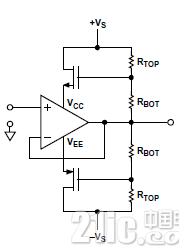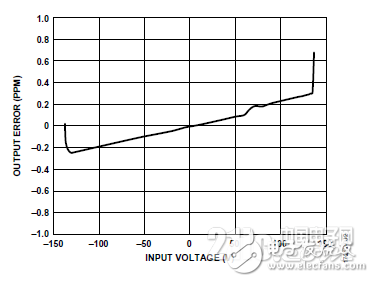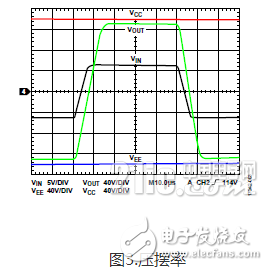# Explain in detail how to use the bootstrap extended op amp to work

When off-the-shelf op amps do not provide the range of signal swings required for a particular application, engineers face two choices: using high-voltage op amps or designing discrete solutions—the cost of both options can be high. For many applications, the third option, bootstrapping, may be a cheaper alternative. In addition to applications where dynamic performance is critical, the design of bootstrap power circuits is fairly straightforward.

Introduction

A conventional op amp requires its input voltage to be within its power rail range. If the input signal may exceed the power rail, the input can be attenuated by a resistor to reduce the input to a level within the power supply range. This is not ideal because it can adversely affect input impedance, noise, and drift. The same power rail also limits the amplifier output, and there is a limit on the size of the closed loop gain to avoid driving the output to saturation.

Therefore, if large signal deviations on the input and/or output are required to be processed, wide power rails and amplifiers that can operate on these rails are required. ADI's 220 V ADHV4702-1 is an excellent choice for this situation, but the bootstrap low-voltage op amp also meets the application requirements. Whether or not to use bootstrapping depends mainly on dynamic requirements and power consumption limits.

Bootstrap creates an adaptive dual supply whose positive and negative voltages are not ground referenced, but are based on the instantaneous value of the output signal, sometimes referred to as the flying rail configuration. In this configuration, the power supply moves up and down with the output voltage (VOUT) of the operational amplifier. Therefore, VOUT is always at the intermediate supply voltage and the supply voltage can move relative to ground. This adaptive dual power supply can be implemented very easily using bootstrap.

In fact, the bootstrap must conform to some guidelines, some of which are trivial, but none of them are particularly troublesome. The following are the most basic guidelines:

• The output load must not be too large.

• The response speed must not be lower than the slew rate of the op amp.

• Must be able to handle the required voltage levels and associated power consumption.

working principle

The flying rail concept refers to the continuous adjustment of the positive and negative power rails so that their voltages are always symmetrical about the output voltage. This way, the output is always within the power supply range.

The circuit architecture includes a pair of complementary discrete transistors and a resistive bias network. The NPN emitter (or the source pin of the N-channel MOSFET) provides VCC, and the PNP emitter (or the source pin of the P-channel MOSFET) is used as the VEE. The transistors are biased so that the required supply voltage appears on the +VS and -VS pins of the amplifier, which are obtained from the high voltage supply through a resistor divider. Figure 1 shows a simplified schematic of the high voltage follower.Figure 1. Simplified high voltage follower schematic

In theory, bootstrap can provide any high signal compliance voltage for any op amp. In fact, the larger the power supply adjustment ratio, the worse the dynamic performance, because the slew rate of the operational amplifier limits the response speed of the power supply to the dynamic signal. When the amplifier is operating at or near the maximum rated supply voltage, the power supply pin is the minimum range that needs to traverse to keep up with the dynamic signal. Other sources of error (such as noise gain) are also reduced when the op amp is operating close to its highest rated supply voltage (see "Sampling a Wide Voltage Swing by Operational Amplifier Bootstrap", EDN Magazine, May 13, 1999) .

Low-frequency and DC applications that do not require power to travel very far (or very fast) are the best candidate for bootstrap. Therefore, a high voltage amplifier can provide better dynamic performance than a low voltage amplifier with comparable dynamic characteristics, especially when both are biased to their respective maximum operating supply voltages and bootstrapped to the same signal range. Bootstrap also affects DC performance, so op amps optimized for both DC accuracy and high voltage provide the best combination of DC and AC performance in a bootstrap configuration.

Design Considerations for Range Extenders Using the ADHV4702-1

The ADHV4702-1 is a precision 220 V operational amplifier. With this device, it is not necessary to bootstrap conventional low-voltage operational amplifiers, and the high-voltage design of the signal range below 220 V is simplified. If your application requires a higher voltage, you can apply bootstrap technology to easily double the circuit's operating range. An example of a 500 V amplifier design based on the ADHV4702-1 is described below.

voltage range

As mentioned above, the range of expander circuits is theoretically infinite, but there are some practical limitations as follows:

• Supply voltage and current rating

• Resistance and field effect transistor (FET) power dissipation

• FET breakdown voltage

DC bias level

First, consider the supply voltage supplied to the amplifier. Any voltage within the rated supply voltage range of the device is valid. However, power consumption is distributed between the amplifier and the FET based on the selected operating voltage. For a given raw supply voltage, the lower the op amp supply voltage, the higher the drain-to-source voltage (VDS) in the FET, and the power dissipation is allocated accordingly. The appropriate op amp supply voltage should be chosen to distribute power between devices in a way that is most beneficial for heat dissipation.

Second, use the following equation to calculate the voltage divider ratio required to reduce the original supply voltage (VRAW) to the amplifier's desired supply voltage (VAMP):

VRAW/VAMP = (RTOP + RBOT)/RBOT

Among them, RTOP is the top resistance and RBOT is the bottom resistance.

For the following example, consider the op amp's nominal supply voltage to be ±100 V. For applications requiring a ±250 V swing range, calculate the partial pressure ratio by:

Partial pressure ratio = 250 V/100 V = 2.5 or 2.5:1

Then, design the resistor divider using a readily available standard value resistor to achieve this partial pressure ratio as closely as possible. Please note that due to the high voltage involved, the power dissipation of the resistor may be higher than expected.

Static power

For the selected resistor value, select the resistor size that will handle the corresponding static power dissipation. Conversely, if the physical size of the resistor is limited, the appropriate resistor value should be chosen to limit the heat dissipation to the rated range.

In this example, RTOP reaches 150 V and RBOT reaches 100 V. Using a 2512 resistor rated at 1/2 watt, the design must limit the power dissipation (V2/R) of each resistor to less than 0.5 W. Calculate the minimum value of each resistor as follows:

RTOP = (150 V) 2 / 0.5 W = 45 kΩ (minimum)

RBOT = (100 V) 2/0.5 W = 20 kΩ (minimum)

Using a higher value resistor (45kΩ) as a limiting factor in power consumption, the RBOT value produces a 2.5:1 voltage divider while observing the static power limit to

RBOT = RTOP/1.5 = 30 kΩ

Its power consumption is (100 V) 2/30 kΩ = 0.33 W.

Instantaneous power consumption

Considering that the instantaneous voltage of the resistor depends on the output voltage of the amplifier and the supply voltage, the voltage on each voltage divider can be as high as 350 V (VCC = 250 V and VOUT = -100 V) at any time in this example. The sinusoidal output waveform produces the same average power dissipation in the VCC and VEE voltage dividers, but any non-zero average output will result in one divider having a higher power dissipation than the other. For a full-scale DC output (or square wave), the instantaneous power dissipation is the maximum power dissipation.

In this example, to maintain instantaneous power consumption below 0.5 W, the sum of the two resistors (RSUM) in each voltage divider must not be less than:

RSUM = (350 V) 2/0.5 W = 245 kΩ

Therefore, when the resistance ratio is 1.5:1 (for a 2.5:1 voltage divider), the minimum values ​​of the individual resistors are as follows:

• RTOP = 147 kΩ

• RBOT = 98 kΩ

FET selection

The breakdown voltage required to withstand the worst-case bias conditions primarily determines the choice of FET; this is understood when the output is saturated such that one FET is at maximum VDS and the other FET is at minimum VDS. In the previous example, the maximum absolute VDS is approximately 300 V, which is the total raw supply voltage (500 V) minus the total supply voltage (200 V) of the amplifier. Therefore, the FET must withstand at least 300 V without being broken down.

Power consumption must be calculated for worst case VDS and operating current, and FETs that specify operation at this power level must be selected.

Next consider the gate capacitance of the FET because it will form a low-pass filter with the bias resistor. FETs with higher breakdown voltages tend to have higher gate capacitances, and the bias resistance is often 100 kΩ, so much gate capacitance is not required to significantly reduce the speed of the circuit. The gate capacitance value is obtained from the manufacturer's data sheet, and the pole frequency formed by the parallel combination of RTOP and RBOT is calculated.

The frequency response of the bias network must always be faster than the input and output signals, otherwise the output of the amplifier may exceed its own power supply range. Temporary deviations from the amplifier's power rail can create a risk of damaging the input, while temporary saturation or limited slew can pose a risk of output distortion. Any of these conditions can cause temporary loss of negative feedback and unpredictable transient behavior, and may even be latched by phase reversal in some op amp architectures.

performance

DC linearity

Figure 2 shows the gain error vs. input voltage (DC linearity) with a gain of 20 and a power supply of ±140 V.Figure 2. Gain error vs. input voltage

Slew rate

Figure 3 shows the slew rate curve with a gain of 20, a power supply of ±140 V, and a measured value of 20.22 V/μs.Power consumption

As mentioned earlier, the breakdown voltage (and associated gate capacitance) and resistance of the FET must also be high when the operating voltage is high. Higher resistance and capacitance values ​​result in reduced bandwidth, and the only available adjustment factor is the resistance value. Reducing the value of the resistor increases the bandwidth, but at the expense of increased power consumption. Space resistance of low resistance and high power is large, which requires more board space. Adding some lead compensation to the RBOT in the form of a capacitor improves the frequency response of the circuit. This capacitor forms a zero with the RBOT and RTOP resistors, canceling the pole formed by the FET gate capacitance. The pole and zero are canceled, so you can choose a higher resistance resistor to reduce DC power consumption.

in conclusion

In applications where higher voltages are required but typical high voltage op amps are not economical, conventional op amps are often bootstrapped. Bootstrap has its advantages and disadvantages. As an alternative, the ADHV4702-1 offers a precision, high performance solution up to 220 V without the need for bootstrap. However, when the signal range is required to exceed 220 V, the device can bootstrap to handle voltages more than twice the nominal signal range while providing higher performance than bootstrap low-voltage amplifiers.